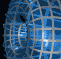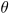Português | English Beyond 3D > Necklaces > Mathematics

# The Mathematics of the Necklaces

Like the z-Squared and z-Cubed tetraviews, the images "z-Squared Necklace" and "z-Cubed Necklace" also show views of the complex squaring and cubing functions. The sequence begins with the graph of the real part of the function (viewed from above, i.e., from the u-axis, so that what we see is just a disc in the xy-plane) and ending with the graph of the real part of the inverse relation (viewed from the negative x-axis, so we see a doubly or triply covered disk in the uv-plane). The intermediate images show views after rotating the surface in both the the xv- and yu-planes by an angle of, for several values ofbetween 0 and 90 degrees. As a projection into 2-space, each view shows a three- or four-fold symmetry. The on-line gallery provides movies that give the complete sequence of which the five in each necklace are a part.

One way to see the symmetry is to look at the boundary of the unit disc in the xy-plane. This can be parameterized as (x, y) = (cos t, sin t). Since we have shown (in the discussion of the tetraviews) that the complex squaring function has the graph (x, y, x2-y2, 2xy), the image of this circle is then

(cos t, sin t, cos2 t - sin2 t, 2 cos t sin t) = (cost, sint, cos 2t, sin 2t).

Rotating this through an angle ofin the xv and yu-planes givesMultiplying the matrices and then taking the orthogonal projection into the xy-plane gives the curve

(x, y) = (coscos t + sinsin 2t, cossin t + sincos 2t),

which equals

(x, y) = cos(cos t, sin t) + sin(sin 2t, cos 2t).

Plotting this curve reveals that it does indeed have the required 3-fold symmetry. it is left as an exercise for the reader to verify that this curve is a hypocycloid formed by a small circle rolling along the inside of a larger circle with radius three times that of the small circle (thus the three-fold symmetry). The point that traces the cycloid may be anywhere along the radius of the small circle (indeed even outside it). In fact, if the radius of the inner circle is normalized to be of unit length, then the point is at a distance of 2 tanfrom the center of the small circle.